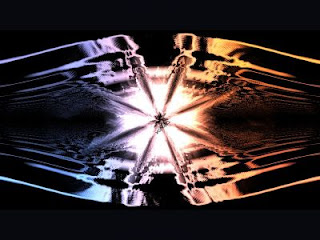## 10/10/07

### Tiny Distortion Shader

Whilst working on Spheres Dream, Pasy of Rebels gave me some code for a distortion shader. I used it to quite good effect but completely size reduced and rewritten. I'll present my distortion shader here but first what is a distortion shader? Well, imagine a lens floating infront of your graphics, it would distort the image behind. To do this in OpenGL we would:
• Draw the image to be distorted to an offscreen buffer
• Capture this to texture (see NPOT article)
• Clear the screen
• Enable a lens distortion shader
• Draw a textured quad
The shader would distort the texture co-ordinates as if looking through a lens. Easy. However the lens can be highly complex, have a bumpy surface or even be a second texture.

The first image is a very flat image before distortion. The second image is applying a distortion shader and adds complexity and interest to the image.I did not want to use a second texture to distort the image. Instead I used a simple trick. The image is drawn and the distort is calculated from the orginal image alone. Infact the distortion is a function of colour in the original image.

Here is the vertex shader:
`const char *vsh="varying vec4 p;\void main(){\gl_Position=ftransform();\p=ftransform();\}";`
So p is set to the co-ordinate of the transformed vertex. Which ranges between -1 and 1 in my screen space.

Here is the fragment shader:
`const char *distortScreenfsh="\uniform sampler2D s;\varying vec4 p;\void main(){\vec4 t=p/2.0+0.5;\float d=length(texture2D(s,t.xy).xyz);\gl_FragColor=texture2D(s,t.xy+d*p.xy*0.3);\}";`
So t maps to between 0..1 and corresponds exactly to (0,0) in one corner of the screen and (1,1) in the opposite corner. This avoids having to define texture co-ordinates in the main OpenGL code and also, infact means one big trick. In the main code now we can use a gluDisk rather than define a real quad! This is far fewer bytes. If I had tried to use real texture co-ordinates in the shader I couldn't use a Disk as the texture co-ordinates would be wrong.

The real magic then is here:
`float d=length(texture2D(s,t.xy).rgb);\gl_FragColor=texture2D(s,t.xy+d*p.xy*0.3);\`
We do a texture lookup in the original image using t as the texture co-ordinates. We read the rgb values and then take the length of this as a vector. So somehow d is a measure of the intensity of colour in the original image.

Then we finally do a texture lookup in the original image, adjusted by d and p. This is the distort right here (+d*p.xy*0.3).

The shader is tiny and produces a much richer feel to the image.